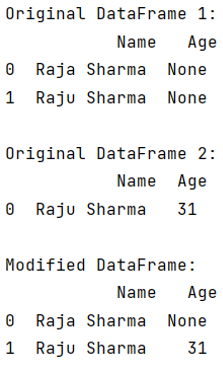# Python - How to update values in a specific row in a Pandas DataFrame?

Given a Pandas DataFrame, we have to update values in a specific row.
Submitted by Pranit Sharma, on August 05, 2022

Pandas is a special tool that allows us to perform complex manipulations of data effectively and efficiently. Inside pandas, we mostly deal with a dataset in the form of DataFrame. DataFrames are 2-dimensional data structures in pandas. DataFrames consist of rows, columns, and data.

Rows in pandas are the different cell (column) values that are aligned horizontally and also provide uniformity. Each row can have the same or different value. Rows are generally marked with the index number but in pandas, we can also assign index names according to the needs.

To learn how to update values in a specific row in a pandas DataFrame, we will select any particular row with the help of the loc[] method, and then we can update the value if it meets a certain condition with another value.

Let us understand with the help of an example,

## Python code to update values in a specific row in a Pandas DataFrame

```# Importing pandas package
import pandas as pd

# Creating a DataFrame
df = pd.DataFrame({
'Name' :  ['Raja Sharma', 'Raju Sharma'],
'Age': [None,None]
})

df2 = pd.DataFrame({
'Name' : ['Raju Sharma'],
'Age':
}, index=)

# Display original DataFrames
print("Original DataFrame 1:\n",df,"\n")

print("Original DataFrame 2:\n",df2,"\n")

# Selecting row and updating value
df.loc[df.Name == 'Raju Sharma', 'Age'] = df2[df2.Name == 'Raju Sharma'].loc['Age']

# Display modified Dataframe
print("Modified DataFrame:\n",df)
```

Output: